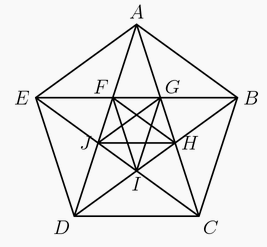###### back to index | new

Define the sequence $a_1, a_2, a_3, \ldots$ by $a_n = \sum\limits_{k=1}^n \sin{k}$, where $k$ represents radian measure. Find the index of the $100^{th}$ term for which $a_n < 0$.

Suppose that the angles of $\triangle ABC$ satisfy $cos(3A)+cos(3B)+cos(3C)=1.$ Two sides of the triangle have lengths $10$ and $13$. There is a positive integer $m$ so that the maximum possible length for the remaining side of $\triangle ABC$ is $\sqrt{m}$. Find $m$.

For $\pi \le \theta < 2\pi$, let\begin{align*} P &= \frac12\cos\theta - \frac14\sin 2\theta - \frac18\cos 3\theta + \frac{1}{16}\sin 4\theta + \frac{1}{32} \cos 5\theta - \frac{1}{64} \sin 6\theta - \frac{1}{128} \cos 7\theta + \cdots \end{align*} and \begin{align*} Q &= 1 - \frac12\sin\theta -\frac14\cos 2\theta + \frac18 \sin 3\theta + \frac{1}{16}\cos 4\theta - \frac{1}{32}\sin 5\theta - \frac{1}{64}\cos 6\theta +\frac{1}{128}\sin 7\theta + \cdots \end{align*} so that $\frac{P}{Q} = \frac{2\sqrt2}{7}$. Then $\sin\theta = -\frac{m}{n}$ where $m$ and $n$ are relatively prime positive integers. Find $m+n$.

Let $A,B,C$ be angles of an acute triangle with $$\cos^2 A + \cos^2 B + 2 \sin A \sin B \cos C = \frac{15}{8}$$ and $$\cos^2 B + \cos^2 C + 2 \sin B \sin C \cos A = \frac{14}{9}$$ There are positive integers $p$, $q$, $r$, and $s$ for which $\cos^2 C + \cos^2 A + 2 \sin C \sin A \cos B = \frac{p-q\sqrt{r}}{s},$ where $p+q$ and $s$ are relatively prime and $r$ is not divisible by the square of any prime. Find $p+q+r+s$.

Let $x$ and $y$ be real numbers such that $\frac{\sin x}{\sin y} = 3$ and $\frac{\cos x}{\cos y} = \frac12$. The value of $\frac{\sin 2x}{\sin 2y} + \frac{\cos 2x}{\cos 2y}$ can be expressed in the form $\frac pq$, where $p$ and $q$ are relatively prime positive integers. Find $p+q$.

Suppose $x$ is in the interval $[0, \frac{\pi}{2}]$ and $\log_{24\sin x} (24\cos x)=\frac{3}{2}$. Find $24\cot^2 x$.

Two circles intersect at points $A$ and $B$. The minor arcs $AB$ measure $30^\circ$ on one circle and $60^\circ$ on the other circle. What is the ratio of the area of the larger circle to the area of the smaller circle?

In $\triangle BAC$, $\angle BAC=40^\circ$, $AB=10$, and $AC=6$. Points $D$ and $E$ lie on $\overline{AB}$ and $\overline{AC}$ respectively. What is the minimum possible value of $BE+DE+CD$?

Find the sum of all the positive solutions of $2\cos2x \left(\cos2x - \cos{\left( \frac{2014\pi^2}{x} \right) } \right) = \cos4x - 1$

The angles in a particular triangle are in arithmetic progression, and the side lengths are $4,5,x$. The sum of the possible values of x equals $a+\sqrt{b}+\sqrt{c}$ where $a, b$, and $c$ are positive integers. What is $a+b+c$?

A geometric sequence $(a_n)$ has $a_1=\sin x$, $a_2=\cos x$, and $a_3= \tan x$ for some real number $x$. For what value of $n$ does $a_n=1+\cos x$?

Let $S$ be the set of all point $(x,y)$ in the coordinate plane such that $0 \le x \le \frac{\pi}{2}$ and $0 \le y \le \frac{\pi}{2}$. What is the area of the subset of $S$ for which $\sin^2x-\sin x \sin y + \sin^2y \le \frac34?$

In the figure shown below, $ABCDE$ is a regular pentagon and $AG=1$. What is $FG + JH + CD$?Let integer $n\ge 2$, prove $$\sin{\frac{\pi}{n}}\cdot\sin{\frac{2\pi}{n}}\cdots\sin{\frac{(n-1)\pi}{n}}=\frac{n}{2^{n-1}}$$

Joel selected an acute angle $x$ (strictly between 0 and 90 degrees) and wrote the value of $\sin x$, $\cos x$, and $\tan x$ on three different cards. Then he gave those cards to three students, Malvina, Paulina, and Georgian, one card to each, and asked them to figure out which trigonometric function (sin, cos, tan) produced their cards. Even after sharing the values on their cards with each other, only Malvian was able to surly identify which function produced the value on her card. Compute the sum of all possible values that Joel wrote on Marlvina's card.

Prove the following identities \begin{align} \sin (3\alpha) &= 4\cdot \sin(60-\alpha)\cdot \sin\alpha\cdot \sin(60+\alpha)\\ \cos (3\alpha) &= 4 \cdot\cos(60-\alpha)\cdot \cos\alpha\cdot \cos(60+\alpha)\\ \tan (3\alpha) &= \tan(60-\alpha) \cdot\tan\alpha \cdot\tan(60+\alpha) \end{align}

Show that $$\sin^2\alpha - \sin^2\beta = \sin(\alpha + \beta)\sin(\alpha-\beta)$$ $$\cos^2\alpha - \cos^2\beta = - \sin(\alpha + \beta)\sin(\alpha-\beta)$$

Compute $$\sin^410^{\circ} +\sin^450^{\circ}+\sin^470^\circ$$

Simplify $$\sin^2\alpha + \sin^2\Big(\alpha + \frac{\pi}{3}\Big)+\sin^2\Big(\alpha - \frac{\pi}{3}\Big)$$

Let $A (x_1, y_1)$, $B (x_2, y_2)$, and $C (x_3, y_3)$ be three points on the unit circle, and $$x_1 + x_2 + x_3 = y_1+y_2+y_3=0$$ Prove $$x_1^2 +x_2^2+x_3^2=y_1^2+y_2^2+y_3^2=\frac{3}{2}$$

Compute $(1+\tan 1^\circ)(1+\tan 2^\circ)\cdots(1+\tan 44^\circ)(1+\tan 45^\circ)$

Compute $$\cos\frac{2\pi}{7} \cdot \cos \frac{4\pi}{7}\cdot \cos \frac{8\pi}{7}$$

Compute $$\cos\frac{\pi}{2n+1}\cdot\cos\frac{2\pi}{2n+1}\cdots\cos\frac{n\pi}{2n+1}$$

Compute $$\Big(1+\cos\frac{\pi}{5}\Big)\Big(1+\cos\frac{3\pi}{5}\Big)$$

Compute $$\sin^2 10^\circ + \cos^2 40^\circ + \sin 10^\circ \cos 40^\circ$$